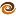# EmptyCrate

#### About Me | Contact Me | Training |ChaiScript.com | YouTube Channel

Say you come up with a clever idea for initializing standard container types (blatantly stolen from the C++0x Initializer List concept). It might looks something like the implementation below:

``````#include <vector>

using namespace std;

template class container, typename DT>
container initializer(const DT &d)
{
container t;
t.insert(t.end(), d);
return t;
}

int main(int, char *[])
{
vector v = initializer(5);
}
``````

But that only works for 1 parameter! Let’s add a second one:

``````#include <vector>

using namespace std;

template class container, typename DT>
container initializer(const DT &d)
{
container t;
t.insert(t.end(), d);
return t;
}

template class container, typename DT>
container initializer(const DT &d, const DT &d2)
{
container t;
t.insert(t.end(), d);
t.insert(t.end(), d2);
return t;
}

int main(int, char *[])
{
vector v = initializer(5, 10);
}
``````

OK, now we have up to two parameters supported. This works… kind of. But it gets tedious VERY quickly and is prone to all kinds of code duplication problems. Using the boost preprocessing library, we can quickly and easily make this code work for 1 to 255 parameters.

``````#include <boost/preprocessor.hpp>

#define rep(z, n, text) t.insert(t.end(), d ## n);

#ifndef BOOST_PP_IS_ITERATING
# ifndef CONTAINER_INITIALIZER_HPP
# define CONTAINER_INITIALIZER_HPP

#define BOOST_PP_ITERATION_LIMITS ( 1, BOOST_PP_LIMIT_ITERATION)

#define BOOST_PP_FILENAME_1 "container_initializer.hpp"
#include BOOST_PP_ITERATE()
# endif // FUSION_MAKE_TUPLE_HPP
#else
# define n BOOST_PP_ITERATION()

template class Container, typename DataType>
Container initializer(
BOOST_PP_ENUM_PARAMS(n, const DataType &d))
{
Container t;
BOOST_PP_REPEAT(n, rep, ~)
return t;
}

#endif
``````

I find this to be a much more practical example than what I was showing in my previous post on this topic. Now, on to the intro to templates. Templates provide a very flexible way of writing generic code. As my test case for the above container initializer, I have written a simple template function that will output a container to standard out. Here is the complete example:

``````#include "container_initializer.hpp"

#include <vector>
#include <list>
#include <string>
#include <set>
#include <iostream>

template
void print_container(OutItr begin, OutItr end)
{
while (begin != end) {
std::cout << *(begin++) << " ";
}

std::cout << std::endl;
}

int main()
{

std::vector v = initializer(5, 16, 43, 27, 192);
std::list l = initializer("hi", "there", "world");
std::set s = initializer("hi","hi","there","world","world");

print_container(v.begin(), v.end());
print_container(l.begin(), l.end());
print_container(s.begin(), s.end());
}
``````

Any questions? The first one I can think of, is, what is there one template parameter in the first example (`initializer(5, 16, 43, 27, 192)`), but two in the second (`initializer("hi", "there", "world")`)? This is because the compiler was trying to create a std::list of const char *, instead of string. I needed to specify the second type when using strings so that the compiler would apply the conversion to string for me.

My Videos

Related